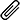# Answering to All Assessments Questions

High Quality, Fast Delivery, Plagiarism Free - Just in 3 Steps

## Submit Your Question HereMultiple Attach File

# Java program to generate a math practice test for a rst grader.

Problem 1 Math Quiz (100%) Write a Java program to generate a math practice test for a rst grader. 1. Generate 100 questions 2. Questions include multiplication, addition, subtraction, and division. 3. First grade math does not teach negative numbers. For subtraction, minuend must be greater than or equal to subtrahend. For division problems, quotient must be an integer. 4. Operands of addition and subtraction are in the range of [1-200]. Operands of multiplication and division are in the range of [1-99]. 5. There is a Question supercl... More

Top